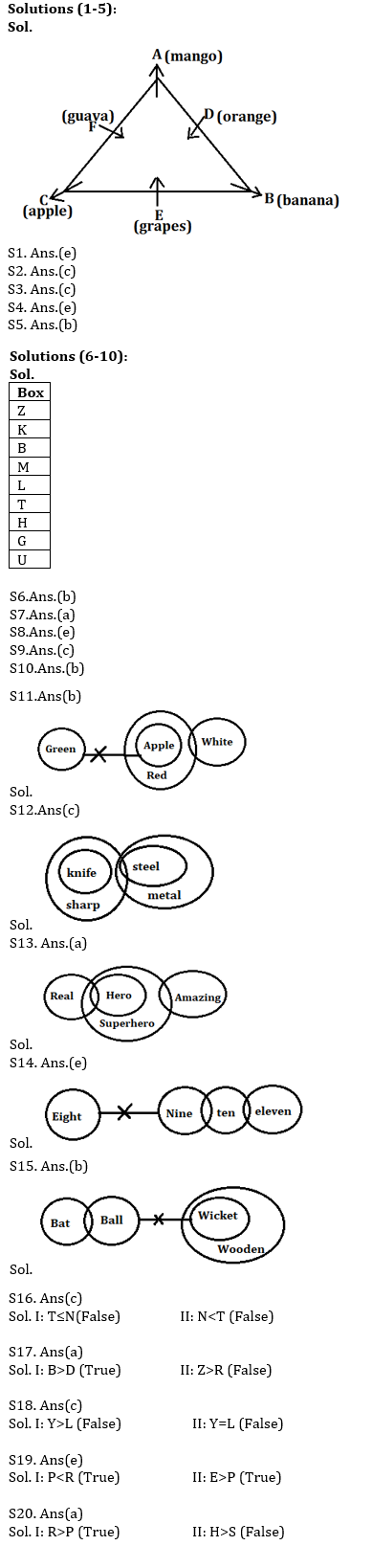Latest Banking jobs   »   Reasoning Ability Quiz For SEBI GRADE...

# Reasoning Ability Quiz For SEBI GRADE A PHASE-I 2022-27th January

Directions (1-5): Study the information carefully and answer the questions given below:
Six persons are sitting around a triangular table such that three of them sit at the corners and the rest on the middle of the side. The one who sits at the corner face away from the center and the one who sits at the middle of the side face towards the center. They also like different fruits. A sits second to the left of the one who likes banana. Only two persons sit between C and D. F sits second to the left of the one who likes grapes. C sits second to the right of B. Neither F nor C likes banana. The one who likes mango sits second to the right of the one who likes apple. D sits middle of the side and likes orange. Neither A nor E likes guava.

Q1. Who among the following likes guava?
(a) B
(b) C
(c) D
(d) E
(e) F

Q2. Who among the following sits immediate right of the one who likes banana?
(a) A
(b) C
(c) The one who likes grapes
(d) The one who likes orange
(e) F

Q3. How many persons sit between A and C, when counted right of A?
(a) None
(b) Two
(c) Three
(d) One
(e) More than three

Q4. What is the position of D with respect to F?
(a) Immediate left
(b) Third to the right
(c) Immediate right
(d) Third to the left
(e) Second to the left

Q5. Four of the following five from a group, which among the following does not belong to this group?
(a) F-Mango
(b) A-Grapes
(c) E-Apple
(d) B-Orange
(e) D-Banana

Directions (6-10): Study the information carefully and answer the questions given below.
Nine boxes are placed one above others in a stack. Four boxes are placed between box H and K. Box K is placed above box H. One box is placed between Box H and L. Three boxes are placed between box M and G. Two boxes are placed between Box U and T. Box Z is placed above box B. Box M is not placed just above or just below box K. Box M is placed above box H. Box K is not placed at topmost of stack. Box B placed just above box M. Box T is not placed at the bottom of the stack.

Q6. Which of the following box is placed just above box L?
(a) B
(b) M
(c) T
(d) H
(e) None of these

Q7. How many boxes are placed in between B and G?
(a) Four
(b) One
(c) Two
(d) Three
(e) None

Q8. Which of the following box is placed above box Z?
(a) One
(b) Two
(c) Four
(d) Three
(e) No one

Q9. Four of the following five are alike in a certain way based form a group, find the one that does not belong to that group?
(a) K-M
(b) B-L
(c) T-U
(d) Z-B
(e) G-T

Q10. If box B and box T interchanged their position then which of the following box is placed just below box B?
(a) M
(b) H
(c) L
(d) G
(e) None of these

Directions (11-15): In each of the questions below are given some statements followed by some Conclusions. You have to take the given statements to be true even, if they seem to be at variance from commonly known facts. Read all the conclusions and then decide which of the given conclusions logically follows from the given statements disregarding commonly known facts.
(a) If only conclusion I follows.
(b) If only conclusion II follows.
(c) If either conclusion I or II follows.
(d) If neither conclusion I nor II follows.
(e) If both conclusions I and II follow.

Q11. Statements:
All apple are red
Some red are white
No apple is green
Conclusions:
I: All white can never be green
II: Some red are not green

Q12. Statements:
All knife is sharp
Some sharp is steel
All steel are metal
Conclusions:
I: Some metal is knife
II: No knife is metal

Q13. Statements:
Some real are hero
All hero are superhero
Some superhero are amazing
Conclusions:
I. Some Real can be amazing
II. Some hero can be real

Q14. Statements:
No Eight is Nine
Some ten are eleven
A few nine are ten
Conclusions:
I. Some ten can be eight
II. All ten can be nine

Q15. Statements:
Some bat are ball
No ball is wicket
All wicket are wooden
Conclusions:
I. Some wooden are not bat
II. All bat can never be wicket

Direction (16-20): In these questions, relationship between different elements is show in the statements. The statements are followed by conclusions. Study the conclusions based on the given statements and select the appropriate answer:
(a) If only conclusion I follows.
(b) If only conclusion II follows.
(c) If either conclusion I or II follows
(d) If neither conclusion I nor II follows.
(e) If both conclusions I and II follow.

Q16.
Statements: P>O, K≥M>T, O=N<J, R<T, J>K
Conclusion I: T≤N II: N<T

Q17.
Statements: T=Y, D<R, U≤Z , X>B>O, Y<R≤U<O
Conclusion I: B>D II: Z>R

Q18.
Statements: H=I≤S ,Y=N, E=L<M , N≥S≥E
Conclusion I: Y>L II: Y=L

Q19.
Statements: P<V<X, E>H, R≥O≥L, H>V<L
Conclusion I: P<R II: E>P

Q20.
Statements: R>T=B, H≥D<S, L≥I=P, L<S<B
Conclusion I: R>P II: H>S

Solutions#### Congratulations!